Next: Exercises Up: One-Dimensional Potentials Previous: Square Potential Well

# Simple Harmonic Oscillator

The classical Hamiltonian of a simple harmonic oscillator is(389)

where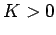is the so-called force constant of the oscillator. Assuming that the quantum mechanical Hamiltonian has the same form as the classical Hamiltonian, the time-independent Schrödinger equation for a particle of massand energymoving in a simple harmonic potential becomes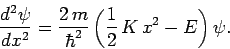(390)

Let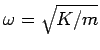, whereis the oscillator's classical angular frequency of oscillation. Furthermore, let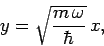(391)

and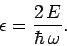(392)

Equation (390) reduces to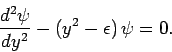(393)

We need to find solutions to the above equation which are bounded at infinity: i.e., solutions which satisfy the boundary condition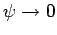as.

Consider the behavior of the solution to Eq. (393) in the limit. As is easily seen, in this limit the equation simplifies somewhat to give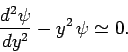(394)

The approximate solutions to the above equation are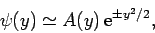(395)

where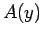is a relatively slowly varying function of. Clearly, ifis to remain bounded asthen we must chose the exponentially decaying solution. This suggests that we should write(396)

where we would expect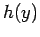to be an algebraic, rather than an exponential, function of.

Substituting Eq. (396) into Eq. (393), we obtain(397)

Let us attempt a power-law solution of the form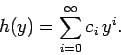(398)

Inserting this test solution into Eq. (397), and equating the coefficients of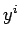, we obtain the recursion relation(399)

Consider the behavior ofin the limit. The above recursion relation simplifies to(400)

Hence, at large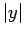, when the higher powers ofdominate, we have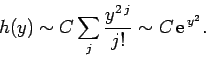(401)

It follows that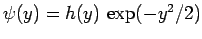varies as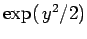as. This behavior is unacceptable, since it does not satisfy the boundary conditionas. The only way in which we can prevent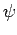from blowing up asis to demand that the power series (398) terminate at some finite value of. This implies, from the recursion relation (399), that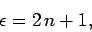(402)

where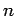is a non-negative integer. Note that the number of terms in the power series (398) is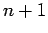. Finally, using Eq. (392), we obtain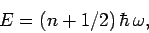(403)

for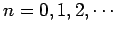.

Hence, we conclude that a particle moving in a harmonic potential has quantized energy levels which are equally spaced. The spacing between successive energy levels is, whereis the classical oscillation frequency. Furthermore, the lowest energy state () possesses the finite energy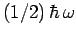. This is sometimes called zero-point energy. It is easily demonstrated that the (normalized) wavefunction of the lowest energy state takes the form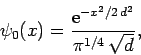(404)

where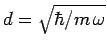.

Letbe an energy eigenstate of the harmonic oscillator corresponding to the eigenvalue(405)

Assuming that the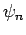are properly normalized (and real), we have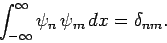(406)

Now, Eq. (393) can be written(407)

where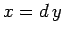, and. It is helpful to define the operators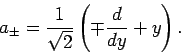(408)

As is easily demonstrated, these operators satisfy the commutation relation(409)

Using these operators, Eq. (407) can also be written in the forms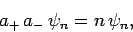(410)

or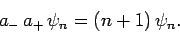(411)

The above two equations imply that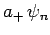(412)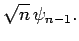(413)

We conclude that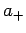andare raising and lowering operators, respectively, for the harmonic oscillator: i.e., operating on the wavefunction withcauses the quantum numberto increase by unity, and vice versa. The Hamiltonian for the harmonic oscillator can be written in the form(414)

from which the result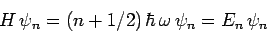(415)

is readily deduced. Finally, Eqs. (406), (412), and (413) yield the useful expression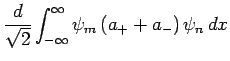(416)SubsectionsNext: Exercises Up: One-Dimensional Potentials Previous: Square Potential Well
Richard Fitzpatrick 2010-07-20